Theory and Modern Applications

# On the hyper exponent of convergence of zeros of ${f}^{\left(j\right)}-\phi$ of higher order linear differential equations

## Abstract

In this paper, we deal with the relationship between the small function and the derivative of solutions of higher order linear differential equations

${f}^{\left(k\right)}+{A}_{k-1}{f}^{\left(k-1\right)}+\cdots +{A}_{0}f=0\phantom{\rule{1em}{0ex}}\left(k\ge 2\right),$

where ${A}_{j}\left(z\right)$ ($j=0,1,\dots ,k-1$) are entire functions or meromorphic functions. The theorems of this paper improve the previous results given by Chen, Belaïdi, Liu.

MSC:34M10, 30D35.

## 1 Introduction and main results

Complex oscillation theory of solutions of linear differential equations in the complex plane $\mathbb{C}$ was started by Bank and Laine [2, 3]. After their well-known work, many important results have been obtained on the complex oscillation theory of solutions of linear differential equations in $\mathbb{C}$, refer to [16, 17].

To state those correlated results, we require to give some explanation as follows.

We shall assume that the reader is familiar with the fundamental results and the standard notations of the Nevanlinna value distribution theory of meromorphic functions (see [11, 14, 16]). In addition, we will use the notation $\sigma \left(f\right)$ to denote the order of meromorphic function $f\left(z\right)$, $\lambda \left(f\right)$ to denote the exponent of convergence of the zero-sequence of $f\left(z\right)$ and $\overline{\lambda }\left(f\right)$ to denote exponent of convergence of distinct zero-sequence of meromorphic function $f\left(z\right)$, and $\tau \left(f\right)$ to denote the type of an entire function $f\left(z\right)$ with $0<\sigma \left(f\right)=\sigma <+\mathrm{\infty }$, which is defined to be (see )

$\tau \left(f\right)=\underset{r\to \mathrm{\infty }}{lim sup}\frac{logM\left(r,f\right)}{{r}^{\sigma }}.$

We use ${\sigma }_{2}\left(f\right)$ to denote the hyper-order of $f\left(z\right)$, ${\sigma }_{2}\left(f\right)$ is defined to be (see )

${\sigma }_{2}\left(f\right)=\underset{r\to \mathrm{\infty }}{lim sup}\frac{loglogT\left(r,f\right)}{logr}.$

We use ${\lambda }_{2}\left(f\right)$ (${\overline{\lambda }}_{2}\left(f\right)$) to denote the hyper-exponent of convergence of the zero-sequence (distinct zero-sequence) of meromorphic function $f\left(z\right)$, ${\lambda }_{2}\left(f\right)$, ${\overline{\lambda }}_{2}\left(f\right)$ are defined to be (see [11, 16])

${\lambda }_{2}\left(f\right)=\underset{r\to \mathrm{\infty }}{lim sup}\frac{loglogN\left(r,\frac{1}{f}\right)}{logr},\phantom{\rule{1em}{0ex}}\text{and}\phantom{\rule{1em}{0ex}}{\overline{\lambda }}_{2}\left(f\right)=\underset{r\to \mathrm{\infty }}{lim sup}\frac{loglog\overline{N}\left(r,\frac{1}{f}\right)}{logr}.$

Let $\phi \left(z\right)$ be an entire function with $\sigma \left(\phi \right)<\sigma \left(f\right)$ or ${\sigma }_{2}\left(\phi \right)<{\sigma }_{2}\left(f\right)$, the hyper-exponent of convergence of zeros and distinct zeros of $f\left(z\right)-\phi \left(z\right)$ are defined to be

${\lambda }_{2}\left(f-\phi \right)=\underset{r\to \mathrm{\infty }}{lim sup}\frac{loglogN\left(r,\frac{1}{f-\phi }\right)}{logr},\phantom{\rule{2em}{0ex}}{\overline{\lambda }}_{2}\left(f-\phi \right)=\underset{r\to \mathrm{\infty }}{lim sup}\frac{loglogN\left(r,\frac{1}{f-\phi }\right)}{logr},$

especially if $\phi \left(z\right)=z$, we use ${\lambda }_{2}\left(f-z\right)$ and ${\overline{\lambda }}_{2}\left(f-z\right)$ to denote the hyper-exponent of convergence of fixed points and distinct fixed points of $f\left(z\right)$, respectively. We use $mE={\int }_{E}dt$ to denote the linear measure of a set $E\subset \left(0,+\mathrm{\infty }\right)$ and use ${m}_{l}E={\int }_{E}\frac{dt}{t}$ to denote the logarithmic measure of a set $E\subset \left[1,+\mathrm{\infty }\right)$. We denote by $S\left(r,f\right)$ any quantity satisfying

$S\left(r,f\right)=o\left(T\left(r,f\right)\right),$

as $r\to +\mathrm{\infty }$, possibly outside of a set with finite measure. A meromorphic function $\psi \left(z\right)$ is called a small function with respect to f if $T\left(r,\psi \right)=S\left(r,f\right)$.

For the second order linear differential equation

${f}^{″}+A\left(z\right){f}^{\prime }+B\left(z\right)f=0,$
(1)

where $A\left(z\right)$ and $B\left(z\right)$ (0) are entire functions, it is an interesting problem to investigate the complex oscillation of solutions of Equation (1). Many mathematicians obtained a lot of important and significant results (see [1, 6, 7, 10, 13]) by studying the above equation.

In 1996, Shon  investigated the hyper-order of the solutions of (1) and obtained the following result.

Theorem A (see )

Let$A\left(z\right)$and$B\left(z\right)$be entire functions such that$\sigma \left(A\right)<\sigma \left(B\right)$or$\sigma \left(B\right)<\sigma \left(A\right)<\frac{1}{2}$, then every function$f\not\equiv 0$of (1) satisfies${\sigma }_{2}\left(f\right)\ge max\left\{\sigma \left(A\right),\sigma \left(B\right)\right\}$.

In 2006, Chen and Shon  investigated the zeros of the solution concerning small functions and fixed points of solutions of second order linear differential equations and obtained some results as follows.

Theorem B (see )

Let${A}_{j}\left(z\right)\not\equiv 0$ ($j=1,2$) be entire functions with$\sigma \left({A}_{j}\right)<1$, suppose that a, b are complex numbers and satisfy$ab\ne 0$and$arga\ne argb$or$a=cb$ ($0). If$\phi \left(z\right)\not\equiv 0$is an entire function of finite order, then every non-trivial solution f of the equation

${f}^{″}+{A}_{1}\left(z\right){e}^{az}{f}^{\prime }+{A}_{2}\left(z\right){e}^{bz}f=0$

satisfies$\overline{\lambda }\left(f-\phi \right)=\overline{\lambda }\left({f}^{\prime }-\phi \right)=\overline{\lambda }\left({f}^{″}-\phi \right)=\mathrm{\infty }$.

Theorem C (see )

Let${A}_{1}\left(z\right)\not\equiv 0$, $\phi \left(z\right)\not\equiv 0$, $Q\left(z\right)$be entire functions with$\sigma \left({A}_{1}\right)<1$, $1<\sigma \left(Q\right)<\mathrm{\infty }$and$\sigma \left(\phi \right)<\mathrm{\infty }$, then every non-trivial solution f of the equation

${f}^{″}+{A}_{1}\left(z\right){e}^{az}{f}^{\prime }+Q\left(z\right)f=0$

satisfies$\overline{\lambda }\left(f-\phi \right)=\overline{\lambda }\left({f}^{\prime }-\phi \right)=\overline{\lambda }\left({f}^{″}-\phi \right)=\mathrm{\infty }$, where$a\ne 0$is a complex number.

In the same year, Liu and Zhang  investigated the fixed points when the coefficients of the equations are meromorphic functions and obtained the following result.

Theorem D (see )

Suppose that$k\ge 2$and$A\left(z\right)$is a transcendental meromorphic function satisfying$\delta \left(\mathrm{\infty },A\right)={\underline{lim}}_{r\to \mathrm{\infty }}\frac{m\left(r,A\right)}{T\left(r,A\right)}=\delta >0$, $\sigma \left(A\right)=\sigma <+\mathrm{\infty }$. Then every meromorphic solution$f\not\equiv 0$of the equation

${f}^{\left(k\right)}+A\left(z\right)f=0$
(2)

satisfies that f and${f}^{\prime },{f}^{″},\dots ,{f}^{\left(k\right)}$all have infinitely many fixed points and$\overline{\lambda }\left({f}^{\left(j\right)}-z\right)=\sigma$ ($j=0,1,\dots ,k$).

For Equation (2), Belaïdi , Belaïdi and El Farissi  investigated the fixed points and the relationship between small functions and differential polynomials of solutions of Equation (2) and obtained some results which improve Theorems D and C.

There naturally arises an interesting subject on the problems of fixed points of solutions of the differential equation

${f}^{\left(k\right)}+{A}_{k-1}{f}^{\left(k-1\right)}+\cdots +{A}_{0}f=0\phantom{\rule{1em}{0ex}}\left(k\ge 2\right),$
(3)

where ${A}_{j}\left(z\right)$ ($j=0,1,\dots ,k-1$) are entire functions.

In this paper, we will deal with the above equation and investigate the relationship between small functions and derivative of solutions of Equation (2) and obtain some theorems which improve the previous results given by Chen, Kwon and etc.

Theorem 1.1 Let${A}_{j}\left(z\right)$, $j=0,1,\dots ,k-1$be entire functions with finite order and satisfy one of the following conditions:

1. (i)

$max\left\{\sigma \left({A}_{j}\right):j=1,2,\dots ,k-1\right\}<\sigma \left({A}_{0}\right)<\mathrm{\infty }$;

2. (ii)

$0<\sigma \left({A}_{k-1}\right)=\cdots =\sigma \left({A}_{1}\right)=\sigma \left({A}_{0}\right)<\mathrm{\infty }$ and $max\left\{\tau \left({A}_{j}\right):j=1,2,\dots ,k-1\right\}={\tau }_{1}<\tau \left({A}_{0}\right)=\tau$,

then for every solution$f\not\equiv 0$of (3) and for any entire function$\phi \left(z\right)\not\equiv 0$satisfying${\sigma }_{2}\left(\phi \right)<\sigma \left({A}_{0}\right)$, we have

${\overline{\lambda }}_{2}\left(f-\phi \right)={\overline{\lambda }}_{2}\left({f}^{\prime }-\phi \right)={\overline{\lambda }}_{2}\left({f}^{″}-\phi \right)={\overline{\lambda }}_{2}\left({f}^{‴}-\phi \right)={\overline{\lambda }}_{2}\left({f}^{\left(i\right)}-\phi \right)={\sigma }_{2}\left(f\right)=\sigma \left({A}_{0}\right)\phantom{\rule{1em}{0ex}}\left(i\in \mathbb{N}\right).$

Theorem 1.2 Let${A}_{j}\left(z\right)$, $j=1,2,\dots ,k-1$be polynomials, ${A}_{0}\left(z\right)$be a transcendental entire function, then for every solution$f\not\equiv 0$of (3) and for any entire function$\phi \left(z\right)$of finite order, we have

1. (i)

$\overline{\lambda }\left(f-\phi \right)=\lambda \left(f-\phi \right)=\sigma \left(f\right)=\mathrm{\infty }$;

2. (ii)

$\overline{\lambda }\left({f}^{\left(i\right)}-\phi \right)=\lambda \left({f}^{\left(i\right)}-\phi \right)=\sigma \left({f}^{\left(i\right)}-\phi \right)=\mathrm{\infty }$ ($i\ge 1,i\in \mathbb{N}$).

Theorem 1.3 Let${A}_{j}\left(z\right)$, $j=0,1,\dots ,k-1$be meromorphic functions satisfying$max\left\{\sigma \left({A}_{j}\right):j=1,2,\dots ,k-1\right\}<\sigma \left({A}_{0}\right)$and$\delta \left(\mathrm{\infty },{A}_{0}\right)>0$. Then for every meromorphic solution$f\not\equiv 0$of (3) and for any meromorphic function$\phi \left(z\right)\not\equiv 0$satisfying${\sigma }_{2}\left(\phi \right)<\sigma \left({A}_{0}\right)$, we have${\overline{\lambda }}_{2}\left({f}^{\left(i\right)}-\phi \right)={\lambda }_{2}\left({f}^{\left(i\right)}-\phi \right)\ge \sigma \left({A}_{0}\right)$ ($i=0,1,\dots$), where${f}^{\left(0\right)}=f$.

Remark 1.1 The following example shows that Theorem 1.3 is not valid when ${A}_{j}\left(z\right)$, $j=0,1,\dots ,k-1$ do not satisfy the condition $max\left\{\sigma \left({A}_{j}\right):j=1,2,\dots ,k-1\right\}<\sigma \left({A}_{0}\right)$.

Example 1.1 For the equation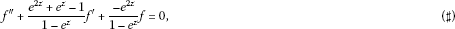we can easily get that Equation (♯) has a solution $f\left(z\right)={e}^{{e}^{z}}+{e}^{z}$. And the functions $\frac{{e}^{2z}+{e}^{z}-1}{1-{e}^{z}}$, $\frac{-{e}^{2z}}{1-{e}^{z}}$ are meromorphic and satisfy $\delta \left(\mathrm{\infty },\frac{-{e}^{2z}}{1-{e}^{z}}\right)=\frac{1}{2}$. Take $\phi \left(z\right)={e}^{z}$, then ${\sigma }_{2}\left(\phi \right)<\sigma \left(\frac{-{e}^{2z}}{1-{e}^{z}}\right)$. Thus, we can get that ${\overline{\lambda }}_{2}\left({f}^{\prime }-\phi \right)={\overline{\lambda }}_{2}\left({e}^{{e}^{z}}{e}^{z}\right)=0\ne 1=\sigma \left(\frac{-{e}^{2z}}{1-{e}^{z}}\right)$.

From Theorems 1.1-1.3, if $\phi \left(z\right)=z$, we can get the following corollaries easily.

Corollary 1.1 Under the assumptions of Theorem 1.1, if$\phi \left(z\right)=z$, for every solution$f\not\equiv 0$of (3), we have

${\overline{\lambda }}_{2}\left(f-z\right)={\overline{\lambda }}_{2}\left({f}^{\prime }-z\right)={\overline{\lambda }}_{2}\left({f}^{″}-z\right)={\overline{\lambda }}_{2}\left({f}^{\left(i\right)}-z\right)={\sigma }_{2}\left(f\right)=\sigma \left({A}_{0}\right)\phantom{\rule{1em}{0ex}}\left(i\in \mathbb{N}\right).$

Corollary 1.2 Under the assumptions of Theorem 1.2, if$\phi \left(z\right)=z$, for every solution$f\not\equiv 0$of (3), we have

1. (i)

$\overline{\lambda }\left(f-z\right)=\lambda \left(f-z\right)=\sigma \left(f\right)=\mathrm{\infty }$;

2. (ii)

$\overline{\lambda }\left({f}^{\left(i\right)}-z\right)=\lambda \left({f}^{\left(i\right)}-z\right)=\sigma \left({f}^{\left(i\right)}-z\right)=\mathrm{\infty }$ ($i\ge 1,i\in \mathbb{N}$).

Corollary 1.3 Under the assumptions of Theorem 1.3, if$\phi \left(z\right)=z$, for every meromorphic solution$f\not\equiv 0$of (3), we have${\overline{\lambda }}_{2}\left({f}^{\left(i\right)}-z\right)={\lambda }_{2}\left({f}^{\left(i\right)}-z\right)\ge {\sigma }_{2}\left({A}_{0}\right)$ ($i=0,1,\dots$), where${f}^{\left(0\right)}=f$.

Remark 1.2 In Theorem B, if $ab\ne 0$ and $a=cb$ ($0), it is easy to see that $\sigma \left({A}_{1}{e}^{az}\right)=\sigma \left({A}_{2}{e}^{bz}\right)=1$ and $\tau \left({A}_{1}{e}^{az}\right)=|a|<\tau \left({A}_{2}{e}^{bz}\right)=|b|$. By Theorem 1.1, for every solution $f\not\equiv 0$ of (3) and for any entire function $\phi \left(z\right)\not\equiv 0$ with ${\sigma }_{2}\left(\phi \right)<1$, we have ${\overline{\lambda }}_{2}\left(f-\phi \right)={\overline{\lambda }}_{2}\left({f}^{\prime }-\phi \right)={\overline{\lambda }}_{2}\left({f}^{″}-\phi \right)=1$. Therefore, Theorem 1.1 is also a partial extension of Theorem B. Theorem 1.2 is the improvement of Theorem C. Theorem 1.3 and Corollary 1.3 are the improvements of Theorem D.

## 2 Some lemmas

To prove our theorems, we require the following lemmas.

Lemma 2.1 Assume$f\not\equiv 0$is a solution of Equation (3), set$g=f-\phi$, then g satisfies the equation

${g}^{\left(k\right)}+{A}_{k-1}{g}^{\left(k-1\right)}+\cdots +{A}_{0}g=-\left[{\phi }^{\left(k\right)}+{A}_{k-1}{\phi }^{\left(k-1\right)}+\cdots +{A}_{0}\phi \right].$
(4)

Proof Since $g=f-\phi$, we have ${g}^{\prime }={f}^{\prime }-{\phi }^{\prime },\dots ,{g}^{\left(k\right)}={f}^{\left(k\right)}-{\phi }^{\left(k\right)}$. Substituting them into Equation (3), we have (4). □

Lemma 2.2 Assume$f\not\equiv 0$is a solution of Equation (3), set${g}_{1}={f}^{\prime }-\phi$, then${g}_{1}$satisfies the equation

${g}_{1}^{\left(k\right)}+{U}_{k-1}^{1}{g}_{1}^{\left(k-1\right)}+\cdots +{U}_{0}^{1}{g}_{1}=-\left[{\phi }^{\left(k\right)}+{U}_{k-1}^{1}{\phi }^{\left(k-1\right)}+\cdots +{U}_{0}^{1}\phi \right],$
(5)

where${U}_{j}^{1}={A}_{j+1}^{\prime }+{A}_{j}-\frac{{A}_{0}^{\prime }}{{A}_{0}}{A}_{j+1}$, $j=0,1,2,\dots ,k-1$and${A}_{k}\equiv 1$.

Proof Since ${g}_{1}={f}^{\prime }-\phi$, we have

${f}^{\prime }={g}_{1}+\phi ,\phantom{\rule{2em}{0ex}}{f}^{″}={g}_{1}^{\prime }+{\phi }^{\prime },\dots ,{f}^{\left(k+1\right)}={g}_{1}^{\left(k\right)}+{\phi }^{\left(k\right)}.$
(6)

And the derivation of (3) is

${f}^{\left(k+1\right)}+{A}_{k-1}{f}^{\left(k\right)}+\left({A}_{k-1}^{\prime }+{A}_{k-2}\right){f}^{\left(k-1\right)}+\cdots +\left({A}_{1}^{\prime }+{A}_{0}\right){f}^{\prime }+{A}_{0}^{\prime }f=0.$
(7)

We can rewrite (3) as

$f=-\frac{1}{{A}_{0}}\left({f}^{\left(k\right)}+{A}_{k-1}{f}^{\left(k-1\right)}+\cdots +{A}_{1}{f}^{\prime }\right).$
(8)

Substituting (8) into (7), we have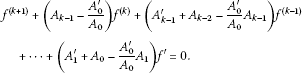(9)

Substituting (6) into (9), we have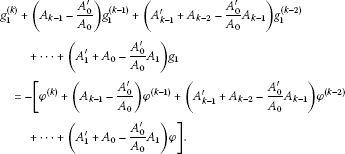(10)

Set ${U}_{j}^{1}={A}_{j+1}^{\prime }+{A}_{j}-\frac{{A}_{0}^{\prime }}{{A}_{0}}{A}_{j+1}$, $j=0,1,2,\dots ,k-1$ and ${A}_{k}\equiv 1$. Then from (9) and (10), we can get

${f}^{\left(k+1\right)}+{U}_{k-1}^{1}{f}^{\left(k\right)}+{U}_{k-2}^{1}{f}^{\left(k-1\right)}+\cdots +{U}_{0}^{1}{f}^{\prime }=0$
(11)

and (5).

Thus, we complete the proof of Lemma 2.2. □

Lemma 2.3 Assume$f\not\equiv 0$is a solution of Equation (3), set${g}_{2}={f}^{″}-\phi$, then${g}_{2}$satisfies the equation

${g}_{2}^{\left(k\right)}+{U}_{k-1}^{2}{g}_{2}^{\left(k-1\right)}+\cdots +{U}_{0}^{2}{g}_{2}=-\left[{\phi }^{\left(k\right)}+{U}_{k-1}^{2}{\phi }^{\left(k-1\right)}+\cdots +{U}_{0}^{2}\phi \right],$
(12)

where${U}_{j}^{2}={{U}_{j+1}^{1}}^{\prime }+{U}_{j}^{1}-\frac{{{U}_{0}^{1}}^{\prime }}{{U}_{0}^{1}}{U}_{j+1}^{1}$, $j=0,1,2,\dots ,k-1$and${U}_{k}^{1}\equiv 1$.

Proof Since ${g}_{2}={f}^{″}-\phi$, we can get

${f}^{″}={g}_{2}+\phi ,\phantom{\rule{2em}{0ex}}{f}^{‴}={g}_{2}^{\prime }+{\phi }^{\prime },\phantom{\rule{2em}{0ex}}\dots ,\phantom{\rule{2em}{0ex}}{f}^{\left(k+2\right)}={g}_{2}^{\left(k\right)}+{\phi }^{\left(k\right)}.$
(13)

The derivation of (11) is

${f}^{\left(k+2\right)}+{U}_{k-1}^{1}{f}^{\left(k+1\right)}+\left({{U}_{k-1}^{1}}^{\prime }+{U}_{k-2}^{1}\right){f}^{\left(k\right)}+\cdots +\left({{U}_{1}^{1}}^{\prime }+{U}_{0}^{1}\right){f}^{″}+{{U}_{0}^{1}}^{\prime }{f}^{\prime }=0.$
(14)

Substituting (11) into (14), we have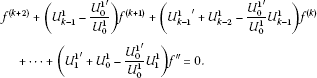(15)

Set ${U}_{j}^{2}={{U}_{j+1}^{1}}^{\prime }+{U}_{j}^{1}-\frac{{{U}_{0}^{1}}^{\prime }}{{U}_{0}^{1}}{U}_{j+1}^{1}$, $j=0,1,2,\dots ,k-1$ and ${U}_{k}^{1}\equiv 1$, then from (14) we have

${f}^{\left(k+2\right)}+{U}_{k-1}^{2}{f}^{\left(k+1\right)}+{U}_{k-2}^{2}{f}^{\left(k\right)}+\cdots +{U}_{0}^{2}{f}^{″}=0.$
(16)

Substituting (13) into (16), we can get (12).

Thus, this completes the proof of Lemma 2.3. □

Lemma 2.4 Assume$f\not\equiv 0$is a solution of Equation (3), set${g}_{3}={f}^{‴}-\phi$, then${g}_{3}$satisfies the equation

${g}_{3}^{\left(k\right)}+{U}_{k-1}^{3}{g}_{3}^{\left(k-1\right)}+\cdots +{U}_{0}^{3}{g}_{3}=-\left[{\phi }^{\left(k\right)}+{U}_{k-1}^{3}{\phi }^{\left(k-1\right)}+\cdots +{U}_{0}^{3}\phi \right],$
(17)

where${U}_{j}^{3}={{U}_{j+1}^{2}}^{\prime }+{U}_{j}^{2}-\frac{{{U}_{0}^{2}}^{\prime }}{{U}_{0}^{2}}{U}_{j+1}^{2}$, $j=0,1,2,\dots ,k-1$and${U}_{k}^{2}\equiv 1$.

Proof Since ${g}_{3}={f}^{‴}-\phi$, we have

${f}^{‴}={g}_{3}+\phi ,\phantom{\rule{2em}{0ex}}{f}^{\left(4\right)}={g}_{3}^{\prime }+{\phi }^{\prime },\phantom{\rule{2em}{0ex}}\dots ,\phantom{\rule{2em}{0ex}}{f}^{\left(k+3\right)}={g}_{3}^{\left(k\right)}+{\phi }^{\left(4\right)}.$
(18)

The derivation of (16) is

${f}^{\left(k+3\right)}+{U}_{k-1}^{2}{f}^{\left(k+2\right)}+\left({{U}_{k-1}^{2}}^{\prime }+{U}_{k-2}^{2}\right){f}^{\left(k+1\right)}+\cdots +\left({{U}_{1}^{2}}^{\prime }+{U}_{0}^{2}\right){f}^{‴}+{{U}_{0}^{2}}^{\prime }{f}^{″}=0.$
(19)

Substituting (16) into (18), we have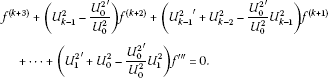(20)

Set ${U}_{j}^{3}={{U}_{j+1}^{2}}^{\prime }+{U}_{j}^{2}-\frac{{{U}_{0}^{2}}^{\prime }}{{U}_{0}^{2}}{U}_{j+1}^{2}$, $j=0,1,2,\dots ,k-1$ and ${U}_{k}^{2}\equiv 1$, then from (20) we have

${f}^{\left(k+3\right)}+{U}_{k-1}^{3}{f}^{\left(k+2\right)}+{U}_{k-2}^{3}{f}^{\left(k+1\right)}+\cdots +{U}_{0}^{3}{f}^{‴}=0.$
(21)

Substituting (18) into (21), we can get (17).

Thus, we complete the proof of Lemma 2.4. □

Lemma 2.5 Assume$f\not\equiv 0$is a solution of Equation (3), set${g}_{i}={f}^{\left(i\right)}-\phi$, then${g}_{i}$satisfies the equation

${g}_{i}^{\left(k\right)}+{U}_{k-1}^{i}{g}_{i}^{\left(k-1\right)}+\cdots +{U}_{0}^{i}{g}_{i}=-\left[{\phi }^{\left(k\right)}+{U}_{k-1}^{i}{\phi }^{\left(k-1\right)}+\cdots +{U}_{0}^{i}\phi \right],$
(22)

where${U}_{j}^{i}={{U}_{j+1}^{i-1}}^{\prime }+{U}_{j}^{i-1}-\frac{{{U}_{0}^{i-1}}^{\prime }}{{U}_{0}^{i-1}}{U}_{j+1}^{i-1}$, $j=0,1,2,\dots ,k-1$, ${U}_{k}^{i-1}\equiv 1$and$i\in \mathbb{N}$.

Proof The inductive method will be used to prove it.

At first, from Lemmas 2.2-2.4, we get that (22) holds for $i=1,2,3$.

Next, suppose that ${g}_{i}={f}^{\left(i\right)}-\phi$, $i\le n$, $n\in \mathbb{N}$ satisfy (22). Thus, ${g}_{n}={f}^{\left(n\right)}-\phi$ satisfies the equation

${g}_{n}^{\left(k\right)}+{U}_{k-1}^{n}{g}_{n}^{\left(k-1\right)}+\cdots +{U}_{0}^{n}{g}_{n}=-\left[{\phi }^{\left(k\right)}+{U}_{k-1}^{n}{\phi }^{\left(k-1\right)}+\cdots +{U}_{0}^{n}\phi \right],$
(23)

where ${U}_{j}^{n}={{U}_{j+1}^{n-1}}^{\prime }+{U}_{j}^{n-1}-\frac{{{U}_{0}^{n-1}}^{\prime }}{{U}_{0}^{n-1}}{U}_{j+1}^{n-1}$, $j=0,1,2,\dots ,k-1$ and ${U}_{k}^{n-1}\equiv 1$.

Since ${g}_{n}={f}^{\left(n\right)}-\phi$, we have

${f}^{\left(n+1\right)}={g}_{n}^{\prime }+{\phi }^{\prime },\phantom{\rule{2em}{0ex}}{f}^{\left(n+2\right)}={g}_{n}^{″}+{\phi }^{″},\phantom{\rule{2em}{0ex}}\dots ,\phantom{\rule{2em}{0ex}}{f}^{\left(k+n\right)}={g}_{n}^{\left(k\right)}+{\phi }^{\left(k\right)}.$
(24)

From (23) and (24), we have

${f}^{\left(k+n\right)}+{U}_{k-1}^{n}{f}^{\left(k+n-1\right)}+{U}_{k-2}^{n}{f}^{\left(k+n-2\right)}+\cdots +{U}_{0}^{n}{f}^{\left(n\right)}=0.$
(25)

Now we will prove that ${g}_{n+1}={f}^{\left(n+1\right)}-\phi$ satisfies (22). Since ${g}_{n+1}={f}^{\left(n+1\right)}-\phi$, we have

${f}^{\left(n+2\right)}={g}_{n+1}^{\prime }+{\phi }^{\prime },\phantom{\rule{2em}{0ex}}{f}^{\left(n+3\right)}={g}_{n+1}^{″}+{\phi }^{″},\phantom{\rule{2em}{0ex}}\dots ,\phantom{\rule{2em}{0ex}}{f}^{\left(n+k+1\right)}={g}_{n+1}^{\left(k\right)}+{\phi }^{\left(k\right)}.$
(26)

The derivation of (25) is

${f}^{\left(k+n+1\right)}+{U}_{k-1}^{n}{f}^{\left(k+n\right)}+\left({{U}_{k-1}^{n}}^{\prime }+{U}_{k-2}^{n}\right){f}^{\left(k+n-1\right)}+\cdots +{{U}_{0}^{n}}^{\prime }{f}^{\left(n\right)}=0.$
(27)

Substituting (25) into (27), we have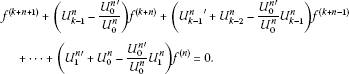(28)

From the definition of ${U}_{j}^{i}$ and (28), we have

${f}^{\left(k+n+1\right)}+{U}_{k-1}^{n+1}{f}^{\left(k+n\right)}+\cdots +{U}_{1}^{n+1}{f}^{\left(n+1\right)}+{U}_{0}^{n+1}{f}^{\left(n\right)}=0,$
(29)

where ${U}_{j}^{n+1}={{U}_{j+1}^{n}}^{\prime }+{U}_{j}^{n}-\frac{{{U}_{0}^{n}}^{\prime }}{{U}_{0}^{n}}{U}_{j+1}^{n}$, $j=0,1,2,\dots ,k-1$ and ${U}_{k}^{n}\equiv 1$. Substituting (26) into (29), we have

${g}_{n+1}^{\left(k\right)}+{U}_{k-1}^{n+1}{g}_{n+1}^{\left(k-1\right)}+\cdots +{U}_{0}^{n+1}{g}_{n+1}=-\left[{\phi }^{\left(k\right)}+{U}_{k-1}^{n+1}{\phi }^{\left(k-1\right)}+\cdots +{U}_{0}^{n+1}\phi \right].$
(30)

This completes the proof of Lemma 2.5. □

Similar to the proof of , Lemma 3.8], we can get the following lemma.

Lemma 2.6 Let$f\left(z\right)$be a transcendental meromorphic function with$\sigma \left(f\right)=\sigma \ge 0$, then there exists a set$E\subset \left[1,+\mathrm{\infty }\right)$with infinite logarithmic measure such that for all$r\in E$, we have

$\underset{r\to \mathrm{\infty }}{lim}\frac{logT\left(r,f\right)}{logr}=\sigma ,\phantom{\rule{1em}{0ex}}r\in E.$

Lemma 2.7 Let${A}_{0}\left(z\right)$, ${A}_{1}\left(z\right)$,…, ${A}_{k-1}\left(z\right)$be entire functions with finite order and satisfy$max\left\{\sigma \left({A}_{j}\right):j=1,2,\dots ,k-1\right\}={\sigma }_{1}<\sigma \left({A}_{0}\right)<\mathrm{\infty }$, and set

${U}_{j}^{1}={A}_{j+1}^{\prime }+{A}_{j}-\frac{{A}_{0}^{\prime }}{{A}_{0}}{A}_{j+1}$

and

${U}_{j}^{i}={{U}_{j+1}^{i-1}}^{\prime }+{U}_{j}^{i-1}-\frac{{{U}_{0}^{i-1}}^{\prime }}{{U}_{0}^{i-1}}{U}_{j+1}^{i-1},$

where$j=0,1,2,\dots ,k-1$, ${A}_{k}\equiv 1$, ${U}_{k}^{i-1}\equiv 1$and$i\in \mathbb{N}$. Then there exists a set E with infinite logarithmic measure such that

$\underset{r\to \mathrm{\infty }}{lim}\frac{logm\left(r,{U}_{0}^{i}\right)}{logr}=\sigma \left({A}_{0}\right)>\underset{r\to \mathrm{\infty }}{lim sup}\frac{{max}_{1\le j\le k-1}\left\{logm\left(r,{U}_{j}^{i}\right)\right\}}{logr}={\sigma }_{1},\phantom{\rule{1em}{0ex}}r\in E.$
(31)

Proof The inductive method will be used to prove it.

First, when $i=1$, i.e., ${U}_{j}^{1}={A}_{j+1}^{\prime }+{A}_{j}-\frac{{A}_{0}^{\prime }}{{A}_{0}}{A}_{j+1}$, $j=0,1,2,\dots ,k-1$ and ${A}_{k}\equiv 1$.

When $j=0$, that is ${U}_{0}^{1}={A}_{1}^{\prime }+{A}_{0}-\frac{{A}_{0}^{\prime }}{{A}_{0}}{A}_{1}$. Then, we have

$m\left(r,{U}_{0}^{1}\right)\le m\left(r,{A}_{1}\right)+m\left(r,{A}_{0}\right)+m\left(r,\frac{{A}_{1}^{\prime }}{{A}_{1}}\right)+m\left(r,\frac{{A}_{0}^{\prime }}{{A}_{0}}\right)+O\left(1\right).$
(32)

From ${A}_{0}=-{A}_{1}^{\prime }+{U}_{0}^{1}+\frac{{A}_{0}^{\prime }}{{A}_{0}}{A}_{1}$, we have

$m\left(r,{A}_{0}\right)\le m\left(r,{A}_{1}\right)+m\left(r,{U}_{0}^{1}\right)+m\left(r,\frac{{A}_{1}^{\prime }}{{A}_{1}}\right)+m\left(r,\frac{{A}_{0}^{\prime }}{{A}_{0}}\right)+O\left(1\right).$
(33)

When $j\ne 0$, $j=1,2,\dots ,k-1$, from the definitions of ${U}_{j}^{1}$, we have

$m\left(r,{U}_{j}^{1}\right)\le m\left(r,{A}_{j+1}\right)+m\left(r,{A}_{j}\right)+m\left(r,\frac{{A}_{0}^{\prime }}{{A}_{0}}\right)+m\left(r,\frac{{A}_{j}^{\prime }}{{A}_{j}}\right)+O\left(1\right).$
(34)

Since ${A}_{j}\left(z\right)$ are entire functions with $max\left\{\sigma \left({A}_{j}\right):j=1,2,\dots ,k-1\right\}<\sigma \left({A}_{0}\right)<\mathrm{\infty }$ and (34), we have

$\underset{1\le j\le k-1}{max}\left\{m\left(r,{U}_{j}^{1}\right)\right\}\le \underset{1\le j\le k-1}{max}\left\{m\left(r,{A}_{j}\right)+o\left(m\left(r,{A}_{0}\right)\right)+O\left(logr\right)\right\}.$
(35)

From (32), (33), (35) and Lemma 2.6, there exists a set $E\subset \left[1,+\mathrm{\infty }\right)$ with infinite logarithmic measure such that

$\begin{array}{rl}\underset{r\to \mathrm{\infty }}{lim}\frac{logm\left(r,{U}_{0}^{1}\right)}{logr}& =\sigma \left({A}_{0}\right)>{\sigma }_{1}=\underset{r\to \mathrm{\infty }}{lim sup}\frac{{max}_{1\le j\le k-1}\left\{logm\left(r,{A}_{j}\right)\right\}}{logr}\\ \ge \underset{r\to \mathrm{\infty }}{lim sup}\frac{{max}_{1\le j\le k-1}\left\{logm\left(r,{U}_{j}^{1}\right)\right\}}{logr},\phantom{\rule{1em}{0ex}}r\in E.\end{array}$
(36)

Now, suppose that (31) holds for $i\le n$, $n\in \mathbb{N}$, that is, there exists a set E with infinite logarithmic measure such that

$\underset{r\to \mathrm{\infty }}{lim}\frac{logm\left(r,{U}_{0}^{n}\right)}{logr}=\sigma \left({A}_{0}\right)>\underset{r\to \mathrm{\infty }}{lim sup}\frac{{max}_{1\le j\le k-1}\left\{logm\left(r,{U}_{j}^{n}\right)\right\}}{logr}={\sigma }_{1}.$
(37)

Next, we prove that (31) holds for $i=n+1$. Since $i=n+1$, we have ${U}_{j}^{n+1}={{U}_{j+1}^{n}}^{\prime }+{U}_{j}^{n}-\frac{{{U}_{0}^{n}}^{\prime }}{{U}_{0}^{n}}{U}_{j+1}^{n}$ where $j=0,1,2,\dots ,k-1$, and ${U}_{k}^{n}\equiv 1$. When $j=0$, When $j=0$, that is ${U}_{0}^{n+1}={{U}_{1}^{n}}^{\prime }+{U}_{0}^{n}-\frac{{{U}_{0}^{n}}^{\prime }}{{U}_{0}^{n}}{U}_{1}^{n}$. Then, we have

$m\left(r,{U}_{0}^{n+1}\right)\le m\left(r,{U}_{0}^{n}\right)+m\left(r,{U}_{1}^{n}\right)+m\left(r,\frac{{{U}_{0}^{n}}^{\prime }}{{U}_{0}^{n}}\right)+m\left(r,\frac{{{U}_{1}^{n}}^{\prime }}{{U}_{1}^{n}}\right)+O\left(1\right).$
(38)

And since ${U}_{0}^{n}={{U}_{1}^{n}}^{\prime }+{U}_{0}^{n+1}-\frac{{{U}_{0}^{n}}^{\prime }}{{U}_{0}^{n}}{U}_{1}^{n}$, we have

$m\left(r,{U}_{0}^{n}\right)\le m\left(r,{U}_{0}^{n+1}\right)+m\left(r,{U}_{1}^{n}\right)+m\left(r,\frac{{{U}_{0}^{n}}^{\prime }}{{U}_{0}^{n}}\right)+m\left(r,\frac{{{U}_{1}^{n}}^{\prime }}{{U}_{1}^{n}}\right)+O\left(1\right).$
(39)

When $j\ne 0$, from the definitions of ${U}_{j}^{n+1}$, $j=1,2,\dots ,k-1$ and ${U}_{k}^{n}\equiv 1$, we have

$m\left(r,{U}_{j}^{n+1}\right)\le m\left(r,{U}_{j+1}^{n}\right)+m\left(r,{U}_{j}^{n}\right)+m\left(r,\frac{{{U}_{j+1}^{n}}^{\prime }}{{U}_{j+1}^{n}}\right)+m\left(r,\frac{{{U}_{0}^{n}}^{\prime }}{{U}_{0}^{n}}\right)+O\left(1\right).$
(40)

From (37)-(40), there exists a set E with infinite logarithmic measure such that

$\begin{array}{rcl}\underset{r\to \mathrm{\infty }}{lim}\frac{logm\left(r,{U}_{0}^{n+1}\right)}{logr}& =& \underset{r\to \mathrm{\infty }}{lim}\frac{logm\left(r,{U}_{0}^{n}\right)}{logr}=\sigma \left({A}_{0}\right)>{\sigma }_{1}\\ =& \underset{r\to \mathrm{\infty }}{lim sup}\frac{{max}_{1\le j\le k-1}\left\{logm\left(r,{U}_{j}^{n}\right)\right\}}{logr}\\ =& \underset{r\to \mathrm{\infty }}{lim sup}\frac{{max}_{1\le j\le k-1}\left\{logm\left(r,{U}_{j}^{n+1}\right)\right\}}{logr}.\end{array}$
(41)

Thus, the proof of Lemma 2.7 is completed. □

Lemma 2.8 Let${H}_{j}\left(z\right)$ ($j=0,1,\dots ,k-1$) be meromorphic functions of finite order. If

$\underset{r\to \mathrm{\infty }}{lim sup}\frac{{max}_{1\le j\le k-1}\left\{logm\left(r,{H}_{j}\right)\right\}}{logr}={\beta }_{1}$

and there exists a set ${E}_{1}$ with infinite logarithmic measure such that

$\underset{r\to \mathrm{\infty }}{lim}\frac{logm\left(r,{H}_{0}\right)}{logr}={\beta }_{2}>{\beta }_{1}$

holds for all$r\in {E}_{1}$, then every meromorphic solution of

${f}^{\left(k\right)}+{H}_{k-1}{f}^{\left(k-1\right)}+\cdots +{H}_{1}{f}^{\prime }+{H}_{0}f=0$
(42)

satisfies${\sigma }_{2}\left(f\right)\ge {\beta }_{2}$.

Proof Assume that $f\left(z\right)$ is a meromorphic solution of (42). From (42), we have

$m\left(r,{H}_{0}\right)\le m\left(r,\frac{{f}^{\left(k\right)}}{f}\right)+m\left(r,\frac{{f}^{\left(k-1\right)}}{f}\right)+\cdots +m\left(r,\frac{{f}^{\prime }}{f}\right)+\sum _{j=1}^{k-1}m\left(r,{H}_{j}\right)+O\left(1\right).$
(43)

By the lemma on logarithmic derivative and (43), we have

$m\left(r,{H}_{0}\right)\le O\left\{logrT\left(r,f\right)\right\}+\sum _{j=1}^{k-1}m\left(r,{H}_{j}\right),\phantom{\rule{1em}{0ex}}r\notin {E}_{2},$
(44)

where ${E}_{2}\subset \left[1,+\mathrm{\infty }\right)$ is a set with finite linear measure. From the assumptions of Lemma 2.6, there exists a set ${E}_{1}$ with infinite logarithmic measure such that for all $|z|=r\in {E}_{1}-{E}_{2}$ we have

${r}^{{\beta }_{2}-\epsilon }\le O\left\{logrT\left(r,f\right)\right\}+\left(k-1\right){r}^{{\beta }_{1}+\epsilon },$
(45)

where $0<2\epsilon <{\beta }_{2}-{\beta }_{1}$. From (45), we have ${\sigma }_{2}\left(f\right)\ge {\beta }_{2}$. □

Lemma 2.9 (see )

Let$f\left(z\right)$be a transcendental meromorphic function with$\sigma \left(f\right)=\sigma <\mathrm{\infty }$, $\mathrm{\Gamma }=\left\{\left({k}_{1},{j}_{1}\right),\dots ,\left({k}_{m},{j}_{m}\right)\right\}$be a finite set of distinct pairs of integers which satisfy${k}_{i}>{j}_{i}\ge 0$for$i=1,\dots ,m$. And let$\epsilon >0$be a given constant, then there exists a set${E}_{3}\subset \left(1,\mathrm{\infty }\right)$that has finite logarithmic measure such that for all z satisfying$|z|=r\notin \left[0,1\right]\cup {E}_{3}$and$\left(k,j\right)\in \mathrm{\Gamma }$, we have

$|\frac{{f}^{\left(k\right)}\left(z\right)}{{f}^{\left(j\right)}\left(z\right)}|\le {|z|}^{\left(k-j\right)\left(\sigma -1+\epsilon \right)}.$

Lemma 2.10 (see )

Let$f\left(z\right)$be an entire function with$\sigma \left(f\right)=\sigma$, $\tau \left(f\right)=\tau$, $0<\sigma <\mathrm{\infty }$, $0<\tau <\mathrm{\infty }$, then for any given$\beta <\tau$, there exists a set${E}_{4}\subset \left[1,+\mathrm{\infty }\right)$that has infinite logarithmic measure such that for all$r\in {E}_{4}$, we have

$logM\left(r,f\right)>\beta {r}^{\sigma }.$

Lemma 2.11 Let${A}_{0}\left(z\right)$, ${A}_{1}\left(z\right)$,…, ${A}_{k-1}\left(z\right)$be entire functions with finite order and satisfy$0<\sigma \left({A}_{0}\right)=\sigma \left({A}_{1}\right)=\cdots =\sigma \left({A}_{k-1}\right)={\sigma }_{2}<\mathrm{\infty }$and$max\left\{\tau \left({A}_{j}\right):j=1,2,\dots ,k-1\right\}={\tau }_{1}<\tau \left({A}_{0}\right)=\tau$, and let${U}_{j}^{1}$, ${U}_{j}^{i}$be stated as in Lemma 2.7, then for any given ε ($0<2\epsilon <\tau -{\tau }_{1}$), there exists a set${E}_{5}$with infinite logarithmic measure such that

$|{U}_{j}^{i}|\le exp\left\{\left({\tau }_{1}+\epsilon \right){r}^{{\sigma }_{2}}\right\},\phantom{\rule{2em}{0ex}}|{U}_{0}^{i}|\ge exp\left\{\left(\tau -\epsilon \right){r}^{{\sigma }_{2}}\right\},$
(46)

where$i\in \mathbb{N}$and$j=1,2,\dots ,k-1$.

Proof The inductive method will be used to prove it.

1. (i)

We first prove that ${U}_{j}^{i}$ ($j=0,1,\dots ,k-1$) satisfy (46) when $i=1$. From the definition of ${U}_{j}^{1}={A}_{j+1}^{\prime }+{A}_{j}-\frac{{A}_{0}^{\prime }}{{A}_{0}}{A}_{j+1}$ ($j\ne 0$) and ${U}_{0}^{1}={A}_{1}^{\prime }+{A}_{0}-\frac{{A}_{0}^{\prime }}{{A}_{0}}{A}_{1}$, we have

$|{U}_{0}^{1}|\ge |{A}_{0}|-|{A}_{1}|\left(|\frac{{A}_{1}^{\prime }}{{A}_{1}}|+|\frac{{A}_{0}^{\prime }}{{A}_{0}}|\right)$
(47)

and

$|{U}_{j}^{1}|\le |{A}_{j}|+|{A}_{j+1}|\left(|\frac{{A}_{j+1}^{\prime }}{{A}_{j+1}}|+|\frac{{A}_{0}^{\prime }}{{A}_{0}}|\right),\phantom{\rule{1em}{0ex}}j=1,2,\dots ,k-1,\phantom{\rule{2em}{0ex}}{A}_{k}\equiv 1.$
(48)

From Lemma 2.9, Lemma 2.10 and (47)-(48), for any ε ($0<4\epsilon <\tau -{\tau }_{1}$), there exists a set ${E}_{5}$ with infinite logarithmic measure such that

$|{U}_{0}^{1}|\ge exp\left\{\left(\tau -\frac{\epsilon }{4}\right){r}^{{\sigma }_{2}}\right\}-2exp\left\{\left({\tau }_{1}+\frac{\epsilon }{4}\right){r}^{{\sigma }_{2}}\right\}{r}^{M}\ge exp\left\{\left(\tau -\frac{\epsilon }{2}\right){r}^{{\sigma }_{2}}\right\}$
(49)

and

$\begin{array}{rl}|{U}_{j}^{1}|& \le exp\left\{\left({\tau }_{1}+\frac{\epsilon }{4}\right){r}^{{\sigma }_{2}}\right\}+2exp\left\{\left({\tau }_{1}+\frac{\epsilon }{4}\right){r}^{{\sigma }_{2}}\right\}{r}^{M}\\ \le exp\left\{\left({\tau }_{1}+\frac{\epsilon }{2}\right){r}^{{\sigma }_{2}}\right\},\phantom{\rule{1em}{0ex}}j\ne 0,\end{array}$
(50)

where $M>0$ is a constant, not necessarily the same at each occurrence.

1. (ii)

Next, we show that ${U}_{j}^{i}$ ($j=0,1,2,\dots ,k-1$) satisfy (46) when $i=2$. From ${U}_{0}^{2}={{U}_{1}^{1}}^{\prime }+{U}_{0}^{1}-\frac{{{U}_{0}^{1}}^{\prime }}{{U}_{0}^{1}}{U}_{1}^{1}$ and ${U}_{j}^{2}={{U}_{j+1}^{1}}^{\prime }+{U}_{j}^{1}-\frac{{{U}_{0}^{1}}^{\prime }}{{U}_{0}^{1}}{U}_{j+1}^{1}$ ($j=0,1,\dots ,k-1$) and ${U}_{k}^{1}\equiv 1$, we have

$|{U}_{0}^{2}|\ge |{U}_{0}^{1}|-|{U}_{1}^{1}|\left(|\frac{{{U}_{1}^{1}}^{\prime }}{{U}_{1}^{1}}|+|\frac{{{U}_{0}^{1}}^{\prime }}{{U}_{0}^{1}}|\right)$
(51)

and

$|{U}_{j}^{2}|\le |{U}_{j}^{1}|+|{U}_{j+1}^{1}|\left(|\frac{{{U}_{j+1}^{1}}^{\prime }}{{U}_{j+1}^{1}}|+|\frac{{{U}_{0}^{1}}^{\prime }}{{U}_{0}^{1}}|\right),\phantom{\rule{1em}{0ex}}j=1,2,\dots ,k-1.$
(52)

By the conclusions of (i) and Lemma 2.9, (49)-(52), for all $|z|=r\in {E}_{5}$,

$|{U}_{0}^{2}|\ge exp\left\{\left(\tau -\frac{\epsilon }{2}\right){r}^{{\sigma }_{2}}\right\}-2exp\left\{\left({\tau }_{1}+\frac{\epsilon }{2}\right){r}^{{\sigma }_{2}}\right\}{r}^{M}\ge exp\left\{\left(\tau -\epsilon \right){r}^{{\sigma }_{2}}\right\}$
(53)

and

$|{U}_{j}^{2}|\le exp\left\{\left({\tau }_{1}+\frac{\epsilon }{2}\right){r}^{{\sigma }_{2}}\right\}+2exp\left\{\left({\tau }_{1}+\frac{\epsilon }{2}\right){r}^{{\sigma }_{2}}\right\}{r}^{M}\le exp\left\{\left({\tau }_{1}+\epsilon \right){r}^{{\sigma }_{2}}\right\},\phantom{\rule{1em}{0ex}}j\ne 0.$
(54)
1. (iii)

Now, suppose that (46) holds for $i\le n$, $n\in \mathbb{N}$, that is, for any given ε ($0<4\epsilon <\tau -{\tau }_{1}$), there exists a set ${E}_{5}$ with infinite logarithmic measure such that

$|{U}_{j}^{i}|\le exp\left\{\left({\tau }_{1}+\epsilon \right){r}^{{\sigma }_{2}}\right\},\phantom{\rule{2em}{0ex}}|{U}_{0}^{i}|\ge exp\left\{\left(\tau -\epsilon \right){r}^{{\sigma }_{2}}\right\}\phantom{\rule{1em}{0ex}}\left(i\le n,j=1,2,\dots ,k-1\right).$
(55)

From ${U}_{0}^{n+1}={{U}_{1}^{n}}^{\prime }+{U}_{0}^{n}-\frac{{{U}_{0}^{n}}^{\prime }}{{U}_{0}^{n}}{U}_{1}^{n}$ and ${U}_{j}^{n+1}={{U}_{j+1}^{n}}^{\prime }+{U}_{j}^{n}-\frac{{{U}_{0}^{n}}^{\prime }}{{U}_{0}^{n}}{U}_{j+1}^{n}$ ($j=0,1,\dots ,k-1$) and ${U}_{k}^{n}\equiv 1$, we have

$|{U}_{0}^{n+1}|\ge |{U}_{0}^{n}|-|{U}_{1}^{n}|\left(|\frac{{{U}_{1}^{n}}^{\prime }}{{U}_{1}^{n}}|+|\frac{{{U}_{0}^{n}}^{\prime }}{{U}_{0}^{n}}|\right)$
(56)

and

$|{U}_{j}^{n+1}|\le |{U}_{j}^{n}|+|{U}_{j+1}^{n}|\left(|\frac{{{U}_{j+1}^{n}}^{\prime }}{{U}_{j+1}^{n}}|+|\frac{{{U}_{0}^{n}}^{\prime }}{{U}_{0}^{n}}|\right),\phantom{\rule{1em}{0ex}}j=1,2,\dots ,k-1.$
(57)

Then, from Lemma 2.9 and (55)-(57), for all $|z|=r\in {E}_{5}$,

$|{U}_{j}^{n+1}|\le exp\left\{\left({\tau }_{1}+\epsilon \right){r}^{{\sigma }_{2}}\right\}+2exp\left\{\left({\tau }_{1}+\epsilon \right){r}^{{\sigma }_{2}}\right\}{r}^{M}\le exp\left\{\left({\tau }_{1}+2\epsilon \right){r}^{{\sigma }_{2}}\right\},\phantom{\rule{1em}{0ex}}j\ne 0$
(58)

and

$|{U}_{0}^{n+1}|\ge exp\left\{\left(\tau -\epsilon \right){r}^{{\sigma }_{2}}\right\}-2exp\left\{\left({\tau }_{1}+\epsilon \right){r}^{{\sigma }_{2}}\right\}{r}^{M}\ge exp\left\{\left(\tau -2\epsilon \right){r}^{{\sigma }_{2}}\right\}.$
(59)

Thus, we complete the proof of Lemma 2.11. □

Lemma 2.12 Let${B}_{j}\left(z\right)$, $j=0,1,\dots ,k-1$be meromorphic functions with$max\left\{\sigma \left({B}_{j}\right):j=1,2,\dots ,k-1\right\}={\sigma }_{4}<\sigma \left({B}_{0}\right)={\sigma }_{3}$and$\delta \left(\mathrm{\infty },{B}_{0}\right)={\underline{lim}}_{r\to \mathrm{\infty }}\frac{m\left(r,{B}_{0}\right)}{T\left(r,{B}_{0}\right)}>0$. Then every meromorphic solution f of the equation

${f}^{\left(k\right)}+{B}_{k-1}{f}^{\left(k-1\right)}+\cdots +{B}_{1}{f}^{\prime }+{B}_{0}f=0$
(60)

satisfies${\sigma }_{2}\left(f\right)\ge {\sigma }_{3}$.

Proof Let f be a meromorphic solution of Equation (60), by (60), we have

$\begin{array}{rl}m\left(r,{B}_{0}\right)& \le m\left(r,\frac{{f}^{\left(k\right)}}{f}\right)+m\left(r,\frac{{f}^{\left(k-1\right)}}{f}\right)+\cdots +m\left(r,\frac{{f}^{\prime }}{f}\right)+\sum _{j=1}^{k-1}m\left(r,{B}_{j}\right)\\ \le O\left\{logrT\left(r,f\right)\right\}+\sum _{j=1}^{k-1}T\left(r,{B}_{j}\right),\phantom{\rule{1em}{0ex}}r\notin {E}_{6},\end{array}$
(61)

where ${E}_{6}\subset \left[1,+\mathrm{\infty }\right)$ is a set with finite linear measure. By Lemma 2.6, there exists a set E with infinite logarithmic measure such that for all $|z|=r\in E$, we have

$\underset{r\to \mathrm{\infty }}{lim}\frac{logT\left(r,{B}_{0}\right)}{logr}={\sigma }_{3},\phantom{\rule{1em}{0ex}}r\notin E.$
(62)

Since $\delta \left(\mathrm{\infty },{B}_{0}\right)>0$, then for any given ε ($0<2\epsilon <{\sigma }_{3}-{\sigma }_{4}$) and for all $r\in E$, by (62), we have

$m\left(r,{B}_{0}\right)\ge {r}^{{\sigma }_{3}-\epsilon }.$
(63)

From (61) and (63), we have

${r}^{{\sigma }_{3}-\epsilon }\le O\left\{logrT\left(r,f\right)\right\}+\left(k-1\right){r}^{{\sigma }_{4}+\epsilon },\phantom{\rule{1em}{0ex}}r\in E-{E}_{6}.$
(64)

From (64), we can get ${\sigma }_{2}\left(f\right)\ge {\sigma }_{3}=\sigma \left({B}_{0}\right)$. □

Lemma 2.13 (see )

Let$f\left(z\right)$be a transcendental meromorphic function and$\alpha >1$be a given constant, for any given$\epsilon >0$, there exists a set${E}_{7}\subset \left[1,\mathrm{\infty }\right)$that has finite logarithmic measure and a constant$M>0$that depends only on α and$\left(m,n\right)$ ($m,n\in \left\{0,\dots ,k\right\}$with$m) such that for all z satisfying$|z|=r\notin \left[0,1\right]\cup {E}_{7}$, we have

$|\frac{{f}^{\left(n\right)}\left(z\right)}{{f}^{\left(m\right)}\left(z\right)}|\le M{\left(\frac{T\left(\alpha r,f\right)}{r}\left({log}^{\alpha }r\right)logT\left(\alpha r,f\right)\right)}^{n-m}.$

Lemma 2.14 Let${B}_{j}\left(z\right)$, $j=0,1,\dots ,k-1$be meromorphic functions of finite order. If there exist positive constants${\sigma }_{5}$, ${\beta }_{3}$, ${\beta }_{4}$ ($0<{\beta }_{3}<{\beta }_{4}$) and a set${E}_{8}$with infinite logarithmic measure such that

$max\left\{|{B}_{j}\left(z\right)|:j=1,2,\dots ,k-1\right\}\le exp\left\{{\beta }_{3}{r}^{{\sigma }_{5}}\right\},\phantom{\rule{1em}{0ex}}|{B}_{0}\left(z\right)|\ge exp\left\{{\beta }_{4}{r}^{{\sigma }_{5}}\right\}$

hold for all$|z|=r\in {E}_{8}$, then every meromorphic solution of (60) satisfies${\sigma }_{2}\left(f\right)\ge {\sigma }_{5}$.

Proof Suppose that f is a meromorphic solution of (60), by (60), we have

$|{B}_{0}\left(z\right)|\le |\frac{{f}^{\left(k\right)}}{f}|+\sum _{j=1}^{k-1}|{B}_{j}\left(z\right)||\frac{{f}^{\left(j\right)}}{f}|.$
(65)

By Lemma 2.13, there exists a set ${E}_{7}$ with finite logarithmic measure such that for all $|z|=r\notin {E}_{7}$, we have

$|\frac{{f}^{\left(j\right)}}{f}|\le M{\left[T\left(2r,f\right)\right]}^{j},\phantom{\rule{1em}{0ex}}j=1,2,\dots ,k,$
(66)

where $M>0$ is a constant. By (65), (66) and the assumptions of Lemma 2.14, for all $|z|=r\in {E}_{8}-{E}_{7}$, we have

$exp\left\{{\beta }_{4}{r}^{{\sigma }_{5}}\right\}\le M{\left[T\left(2r,f\right)\right]}^{k}exp\left\{{\beta }_{3}{r}^{{\sigma }_{5}}\right\}.$
(67)

Since $0<{\beta }_{3}<{\beta }_{4}$, by (67), we have ${\sigma }_{2}\left(f\right)\ge {\sigma }_{5}$. □

Lemma 2.15 (see )

Let${A}_{0},{A}_{1},\dots ,{A}_{k-1},F\not\equiv 0$be meromorphic functions, if f is a meromorphic solution of the equation

${f}^{\left(k\right)}+{A}_{k-1}{f}^{\left(k-1\right)}+\cdot \cdot \cdot +{A}_{0}f=F,$

then we have the following statements:

1. (i)

if $max\left\{\sigma \left(F\right),\sigma \left({A}_{j}\right);j=0,1,\dots ,k-1\right\}<\sigma \left(f\right)=\sigma \le \mathrm{\infty }$, then $\sigma \left(f\right)=\lambda \left(f\right)=\overline{\lambda }\left(f\right)$;

2. (ii)

if $max\left\{{\sigma }_{2}\left(F\right),{\sigma }_{2}\left({A}_{j}\right);j=0,1,\dots ,k-1\right\}<{\sigma }_{2}\left(f\right)=\sigma$, then ${\sigma }_{2}\left(f\right)={\lambda }_{2}\left(f\right)={\overline{\lambda }}_{2}\left(f\right)$.

Lemma 2.16 (see , Theorem 1])

Let${A}_{j}\left(z\right)$ ($j=0,1,\dots ,k-1$) be entire functions satisfying$0<\sigma \left({A}_{k-1}\right)=\cdots =\sigma \left({A}_{1}\right)=\sigma \left({A}_{0}\right)<\mathrm{\infty }$, $max\left\{\tau \left({A}_{j}\right):j=1,2,\dots ,k-1\right\}<\tau \left({A}_{0}\right)<\mathrm{\infty }$, then every solution$f\not\equiv 0$of (3) satisfies${\sigma }_{2}\left(f\right)=\sigma \left({A}_{0}\right)$.

## 3 Proofs of theorems

### 3.1 The proof of Theorem 1.1

To prove the conclusions of Theorem 1.1, we will consider two cases as follows.

Case 1. Suppose that $max\left\{\sigma \left({A}_{j}\right):j=1,2,\dots ,k-1\right\}<\sigma \left({A}_{0}\right)<\mathrm{\infty }$.

1. (i)

First, we prove that ${\overline{\lambda }}_{2}\left(f-\phi \right)={\sigma }_{2}\left(f\right)$. Assume that $f\not\equiv 0$ is a solution of (3), from ref. , we have ${\sigma }_{2}\left(f\right)=\sigma \left({A}_{0}\right)$. Set $g=f-\phi$. Since ${\sigma }_{2}\left(\phi \right)<\sigma \left({A}_{0}\right)$, then ${\sigma }_{2}\left(g\right)={\sigma }_{2}\left(f\right)=\sigma \left({A}_{0}\right)$ and ${\overline{\lambda }}_{2}\left(g\right)={\overline{\lambda }}_{2}\left(f-\phi \right)$. By Lemma 2.1, we get that g satisfies Equation (4). Set $F={\phi }^{\left(k\right)}+{A}_{k-1}{\phi }^{\left(k-1\right)}+\cdots +{A}_{0}\phi$. If $F\equiv 0$, from ref. , we have ${\sigma }_{2}\left(\phi \right)=\sigma \left({A}_{0}\right)$, we can get a contradiction. Then $F\not\equiv 0$, by Lemma 2.15, we have ${\overline{\lambda }}_{2}\left(g\right)={\lambda }_{2}\left(g\right)={\sigma }_{2}\left(g\right)=\sigma \left({A}_{0}\right)$. Thus, we have ${\overline{\lambda }}_{2}\left(f-\phi \right)={\lambda }_{2}\left(f-\phi \right)={\sigma }_{2}\left(f\right)=\sigma \left({A}_{0}\right)$.

2. (ii)

Second, we prove that ${\overline{\lambda }}_{2}\left({f}^{\prime }-\phi \right)={\sigma }_{2}\left(f\right)$. Set ${g}_{1}={f}^{\prime }-\phi$, then ${\sigma }_{2}\left({g}_{1}\right)={\sigma }_{2}\left(f\right)=\sigma \left({A}_{0}\right)$. From Lemma 2.2, we get that ${g}_{1}$ satisfies Equation (5). Set ${F}_{1}={\phi }^{\left(k\right)}+{U}_{k-1}^{1}{\phi }^{\left(k-1\right)}+\cdots +{U}_{0}^{1}\phi$, where ${U}_{j}^{1}$ ($j=0,1,\dots ,k-1$) are stated as in Lemma 2.2. If ${F}_{1}\equiv 0$, from Lemma 2.7 and Lemma 2.8, we have ${\sigma }_{2}\left(\phi \right)\ge \sigma \left({A}_{0}\right)$, a contradiction with ${\sigma }_{2}\left(\phi \right)<\sigma \left({A}_{0}\right)$. Hence ${F}_{1}\not\equiv 0$. By Lemma 2.15, we get ${\overline{\lambda }}_{2}\left({f}^{\prime }-\phi \right)={\lambda }_{2}\left({f}^{\prime }-\phi \right)={\sigma }_{2}\left(f\right)$.

3. (iii)

Now, we prove that ${\overline{\lambda }}_{2}\left({f}^{″}-\phi \right)={\sigma }_{2}\left(f\right)$. Set ${g}_{2}={f}^{″}-\phi$, then ${\sigma }_{2}\left({g}_{2}\right)={\sigma }_{2}\left(f\right)=\sigma \left({A}_{0}\right)$. From Lemma 2.3, we get that ${g}_{2}$ satisfies Equation (12). Set ${F}_{2}={\phi }^{\left(k\right)}+{U}_{k-1}^{2}{\phi }^{\left(k-1\right)}+\cdots +{U}_{0}^{2}\phi$, where ${U}_{j}^{2}$ ($j=0,1,\dots ,k-1$) are stated as in Lemma 2.3. If ${F}_{2}\equiv 0$, from Lemma 2.7 and Lemma 2.8, we have ${\sigma }_{2}\left(\phi \right)\ge \sigma \left({A}_{0}\right)$, a contradiction with ${\sigma }_{2}\left(\phi \right)<\sigma \left({A}_{0}\right)$. Hence ${F}_{2}\not\equiv 0$. By Lemma 2.15, we get ${\overline{\lambda }}_{2}\left({f}^{″}-\phi \right)={\lambda }_{2}\left({f}^{″}-\phi \right)={\sigma }_{2}\left(f\right)$.

4. (iv)

Set ${g}_{3}={f}^{‴}-\phi$. From Lemmas 2.4, 2.7, 2.8 and Lemma 2.15, using the same argument as in Case 1(iii), we can get ${\overline{\lambda }}_{2}\left({f}^{‴}-\phi \right)={\lambda }_{2}\left({f}^{‴}-\phi \right)={\sigma }_{2}\left(f\right)$ easily.

5. (v)

We will prove that ${\overline{\lambda }}_{2}\left({f}^{\left(i\right)}-\phi \right)={\sigma }_{2}\left(f\right)$ ($i>3,i\in \mathbb{N}$). Set ${g}_{i}={f}^{\left(i\right)}-\phi$, then ${\sigma }_{2}\left({g}_{i}\right)={\sigma }_{2}\left(f\right)=\sigma \left({A}_{0}\right)$. From Lemma 2.5, we have ${g}_{i}$ satisfies Equation (22). Set ${F}_{i}={\phi }^{\left(k\right)}+{U}_{k-1}^{i}{\phi }^{\left(k-1\right)}+\cdots +{U}_{0}^{i}\phi$, where ${U}_{j}^{i}$ ($j=0,1,\dots ,k-1;i\in \mathbb{N}$) are stated as in Lemma 2.5. If ${F}_{i}\equiv 0$, from Lemma 2.7 and Lemma 2.8, we have ${\sigma }_{2}\left(\phi \right)\ge \sigma \left({A}_{0}\right)$, a contradiction with ${\sigma }_{2}\left(\phi \right)<\sigma \left({A}_{0}\right)$. Hence ${F}_{i}\not\equiv 0$. By Lemma 2.15, we get ${\overline{\lambda }}_{2}\left({f}^{\left(i\right)}-\phi \right)={\lambda }_{2}\left({f}^{\left(i\right)}-\phi \right)={\sigma }_{2}\left(f\right)$.

Case 2. Suppose that $0<\sigma \left({A}_{k-1}\right)=\cdots =\sigma \left({A}_{1}\right)=\sigma \left({A}_{0}\right)<\mathrm{\infty }$ and $max\left\{\tau \left({A}_{j}\right):j=1,2,\dots ,k-1\right\}={\tau }_{1}<\tau \left({A}_{0}\right)=\tau$.

1. (i)

We first prove that ${\overline{\lambda }}_{2}\left(f-\phi \right)={\sigma }_{2}\left(f\right)$. Assume that $f\not\equiv 0$ is a solution of (3), by Lemma 2.16, we have ${\sigma }_{2}\left(f\right)=\sigma \left({A}_{0}\right)>0$. Set $g=f-\phi$. Since $\phi \not\equiv 0$ is an entire function satisfying ${\sigma }_{2}\left(\phi \right)<\sigma \left({A}_{0}\right)$, then we have ${\sigma }_{2}\left(g\right)={\sigma }_{2}\left(f\right)=\sigma \left({A}_{0}\right)$ and ${\overline{\lambda }}_{2}\left(g\right)={\overline{\lambda }}_{2}\left(f-\phi \right)$. From Lemma 2.1, we get that g satisfies Equation (4). We will affirm $F\not\equiv 0$. If $F\equiv 0$, by Lemma 2.16, we get ${\sigma }_{2}\left(\phi \right)={\sigma }_{2}\left({A}_{0}\right)$, a contradiction. Hence $F\not\equiv 0$. From the assumptions of Theorem 1.1, we get

$max\left\{{\sigma }_{2}\left(F\right),{\sigma }_{2}\left({A}_{j}\right):j=0,1,\dots ,k-1\right\}<{\sigma }_{2}\left(g\right)=\sigma \left({A}_{0}\right).$

From Lemma 2.15(ii), we have ${\overline{\lambda }}_{2}\left(f-\phi \right)={\lambda }_{2}\left(f-\phi \right)={\sigma }_{2}\left(f\right)=\sigma \left({A}_{0}\right)$.

1. (ii)

Now we prove that ${\overline{\lambda }}_{2}\left({f}^{\prime }-\phi \right)={\sigma }_{2}\left(f\right)$. Let ${g}_{1}={f}^{\prime }-\phi$. Since ${\sigma }_{2}\left(\phi \right)<\sigma \left({A}_{0}\right)$, we have ${\sigma }_{2}\left({g}_{1}\right)={\sigma }_{2}\left(f\right)=\sigma \left({A}_{0}\right)$. By Lemma 2.2, we have ${g}_{1}$ satisfies Equation (5). If ${F}_{1}\equiv 0$, from Lemma 2.11 and Lemma 2.14, we have ${\sigma }_{2}\left(\phi \right)\ge \sigma \left({A}_{0}\right)$. Then we can get a contradiction with ${\sigma }_{2}\left(\phi \right)<\sigma \left({A}_{0}\right)$. Therefore, we have ${F}_{1}\not\equiv 0$. By (5) and Lemma 2.15, we have ${\overline{\lambda }}_{2}\left({f}^{\prime }-\phi \right)={\lambda }_{2}\left({f}^{\prime }-\phi \right)={\sigma }_{2}\left(f\right)=\sigma \left({A}_{0}\right)$.

Similar to the arguments as in Case 1(iii)-(v) and by using Lemmas 2.3-2.5, 2.11 and Lemma 2.14, we can get

${\overline{\lambda }}_{2}\left({f}^{\left(i\right)}-\phi \right)={\lambda }_{2}\left({f}^{\left(i\right)}-\phi \right)={\sigma }_{2}\left(f\right)=\sigma \left({A}_{0}\right)\phantom{\rule{1em}{0ex}}\left(i\in \mathbb{N}\right).$

Thus, the proof of Theorem 1.1 is completed.

### 3.2 The proof of Theorem 1.2

Since ${A}_{j}\left(z\right)$ ($j=1,2,\dots ,k-1$) are polynomials and ${A}_{0}\left(z\right)$ is a transcendental entire function, then we have that ${A}_{j}\left(z\right)$ ($j=0,1,2,\dots ,k-1$) satisfy the condition of Theorem 1.1. By using the same argument as in Theorem 1.1 and Lemma 2.15(i), we can get the conclusions of Theorem 1.2 easily.

Thus, we omit the process of proving Theorem 1.2.

### 3.3 The proof of Theorem 1.3

According to the conditions of Theorem 1.3, we can easily get the conclusions of Theorem 1.3 by using the similar argument as in Theorem 1.1 and Lemma 2.15.

## References

1. Amemmiya I, Ozawa M:Non-existence of finite order solutions of ${\omega }^{″}+{e}^{-z}{\omega }^{\prime }+Q\left(z\right)\omega =0$. Hokkaido Math. J. 1981, 10: 1–17.

2. Bank S, Laine I: On the oscillation theory of ${f}^{″}+Af=0$ where A is entire. Trans. Am. Math. Soc. 1982, 273: 351–363.

3. Bank S, Laine I: On the zeros of meromorphic solutions of second order linear differential equations. Comment Math. Helv. 1983, 58: 656–677. 10.1007/BF02564659

4. Belaïdi B: Growth and oscillation theory of solutions of some linear differential equations. Mat. Vesn. 2008, 60(4):233–246.

5. Belaïdi B, El Farissi A: Differential polynomials generated by some complex linear differential equations with meromorphic coefficients. Glas. Mat. 2008, 43(2):363–373. 10.3336/gm.43.2.09

6. Chen ZX: On the hyper order of solutions of some second order linear differential equations. Acta Math. Sin. Engl. Ser. 2002, 18(1):79–88. 10.1007/s101140100107

7. Chen ZX:The growth of solutions of the differential equation ${f}^{″}+{e}^{-z}{f}^{\prime }+Q\left(z\right)f=0$. Sci. China Ser. A 2001, 31: 775–784. in Chinese

8. Chen ZX, Shon KH: The relation between solutions of a class of second order differential equation with functions of small growth. Chin. Ann. Math., Ser. A 2006, 27(A4):431–442. Chinese

9. Chen ZX, Yang CC: Quantitative estimations on the zeros and growths of entire solutions of linear differential equations. Complex Var. Elliptic Equ. 2000, 42: 119–133. 10.1080/17476930008815277

10. Frei M:Uber die subnormalen losungen der differentialgeichung ${\omega }^{″}+{e}^{-z}{\omega }^{\prime }+\left(konst.\right)\omega =0$. Comment. Math. Helv. 1962, 36: 1–8. 10.1007/BF02566887

11. Gao SA, Chen ZX, Chen TW: Oscillation Theory on Linear Differential Equations. Press in Central China Institute of Technology, Wuhan; 1997.

12. Gundersen GG: Estimates for the logarithmic derivative of a meromorphic function, plus similar estimates. J. Lond. Math. Soc. 1988, 305(2):88–104.

13. Gundersen GG:On the question of whether ${f}^{″}+{e}^{-z}{f}^{\prime }+B\left(z\right)f=0$ can admit a solution $f\not\equiv 0$ of finite order. Proc. R. Soc. Edinb., Sect. A, Math. 1986, 102: 9–17. 10.1017/S0308210500014451

14. Hayman W: Meromorphic Functions. Clarendon, Oxford; 1964.

15. Laine I: Nevanlinna Theory and Complex Differential Equations. de Gruyter, Berlin; 1993.

16. Laine I: Complex differential equations. 4. In Handbook of Differential Equations: Ordinary Differential Equations. North-Holland, Amsterdam; 2008:269–363.

17. Liu MS, Zhang XM: Fixed points of meromorphic solutions of higher order linear differential equations. Ann. Acad. Sci. Fenn., Ser. A 1 Math. 2006, 31: 191–211.

18. Shon KH: On the growth of entire functions satisfying second order linear differential equation. Bull. Korean Math. Soc. 1996, 3: 487–496.

19. Tu J, Long T: Oscillation of complex high order linear differential equations with coefficients of finite iterated order. Electron. J. Qual. Theory Differ. Equ. 2009, 66: 1–13.

20. Tu J, Yi CF: On the growth of solutions of a class of higher order linear differential equations with coefficients having the same order. J. Math. Anal. Appl. 2008, 340: 487–497. 10.1016/j.jmaa.2007.08.041

21. Yi HX, Yang CC: Uniqueness Theory of Meromorphic Functions. Science Press/Kluwer Academic, Beijing/New York; 1995/2003.

## Acknowledgements

The authors thank the referee for his/her valuable suggestions to improve the present article.

This work was supported by the Natural Science Foundation of Jiang-Xi Province in China (Grant No. 2009GQS0013 and No. 2010GQS0119) and the Youth Foundation of Education Bureau of Jiangxi Province in China (Grant No. GJJ11072).

## Author information

Authors

### Corresponding author

Correspondence to Hong-Yan Xu.

### Competing interests

The authors declare that they have no competing interests.

### Authors’ contributions

HYX completed the main part of this article, HYX, JT and XMZ corrected the main theorems. All authors read and approved the final manuscript.

## Rights and permissions

Reprints and Permissions

Xu, HY., Tu, J. & Zheng, XM. On the hyper exponent of convergence of zeros of ${f}^{\left(j\right)}-\phi$ of higher order linear differential equations. Adv Differ Equ 2012, 114 (2012). https://doi.org/10.1186/1687-1847-2012-114

• Accepted:

• Published:

• DOI: https://doi.org/10.1186/1687-1847-2012-114

### Keywords

• linear differential equation
• hyper order
• type
• small function